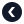Python专题Python 注释

2021-07-02

单行注释

# total是总价
total = 230

total = 230 # total是总价

# total: 总价
# 用于计算购物车内全部商品的总价
total = 230

"""total: 总价

total = 230

'''total: 总价

total = 230

文档注释

Python允许我们为函数方法以及模块编写文档注释。文档注释的书写也是采用的多行注释的风格。 但文档注释的书写位置必须放在紧接着函数方法以及模块定义的下一行。

def calcTotal():
'''计算商品总价'''
total = 30

calcTotal.__doc__

小练习